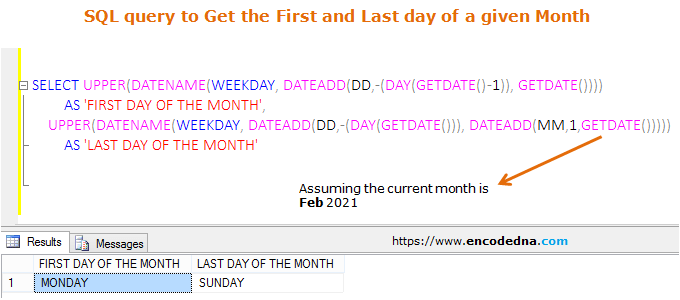﻿ Get the First and Last Day of a given Month in SQL Server

# Get the First and Last Day of a given Month in SQL Server

In SQL Server, you can use the DATENAME() function to get the week day for a given date. I’ll show you how to use this function to get first and last day of a given month or date in SQL Server.

Before we see the query, let us quickly understand the DATENAME() function, how to it and what this function returns.

## Syntax DATENAME() function

DATENAME(interval, date)

The function takes two parameters, an interval and a date. The return type is nvarchar. Its case sensitive.

And, this is how you can use the function in your query.

`SELECT DATENAME(WEEKDAY, GETDATE())`

The output of this query will be the weekday of the current date. And, it should return a value like Monday, Tuesday etc.

Remember: The date can be any date. The GETDATE() will return the current date. You can provide other date like this.

```DECLARE @myDate DATETIME = '02/15/2020';	 -- its mm/dd/yyyy format.
SELECT DATENAME(WEEKDAY, @myDate)```

Instead of WEEKDAY inside the function, you can use W. For example,

`SELECT DATENAME(W, GETDATE())`

The result is the same.

Now we know how to get the weekday of a given date (in words). Let’s see how to use the above function to get the first and last day of a given month or date.

## Get the First and Last Day of a Month

See these two queries.

```SELECT DATEADD(DD,-(DAY(GETDATE() -1)), GETDATE()) AS FirstDate

Executing the above queries will return the first and last day with date, month, year, time etc. For example, if its any day in the month of feb 2021, the output will be …

### FirstDate

2021-02-01 10:44:27.557

### LastDate

2021-02-28 10:44:27.557

However, we want the First and Last day (in words). So, simply embed the two above queries inside the DATENAME() function. Here it is.

```SELECT DATENAME(WEEKDAY, DATEADD(DD,-(DAY(GETDATE() -1)), GETDATE())) AS FirstDay